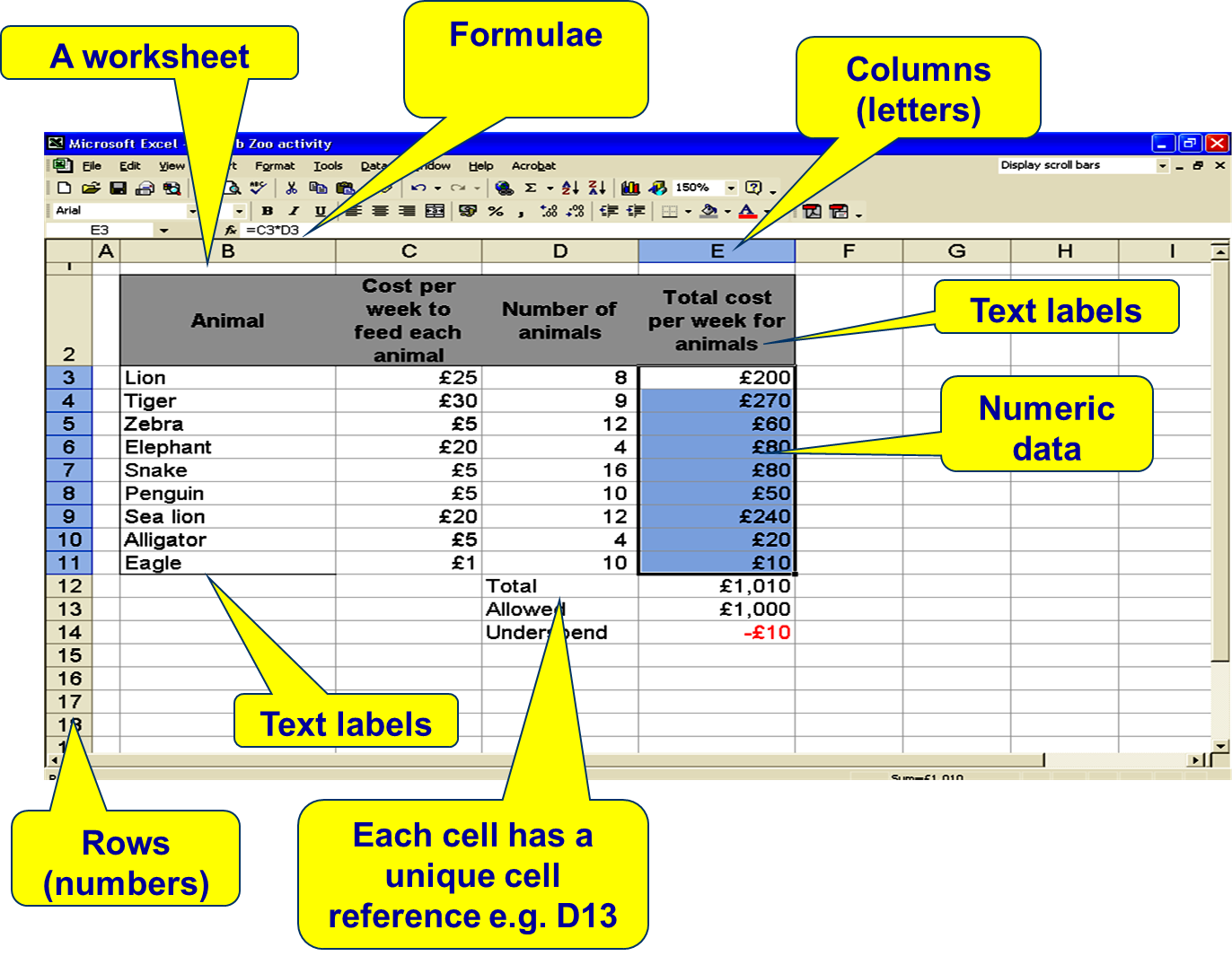Computer Science LearnITWithMrC ⛯ Year 7 Year 8 Year 9 GCSE
0 results for
ICT# Lesson 1 - Formatting and Formula

0 results forGuest
##### Overview##### Learning Objectives
0 results forGuest
IDSkill_name
1 I can calculate sums using a spreadsheet.
3 I can identify the different elements of a spreadsheet e.g. 'row', 'column', 'cell' etc.
2 I can adjust cell height/width and edit columns and rows

#### 🏁 Learning Objective 1 :- I can calculate sums using a spreadsheet.## Calculating Sums

### Extension activity

Put the above sums into an excel worksheet.

See if you can use a simple formula to work them out?#### 🏁 Learning Objective 2 :- I can identify the different elements of a spreadsheet e.g. 'row', 'column', 'cell' etc.A spreadsheet appears as a grid - each row has its own number and each column its own letter. This labelling of rows and columns is used to give each cell a cell address or reference, for example, C5 means column C, row 5.

It is also possible to refer to a range of cells collectively, ie E4:E12 includes E4, E12 and all of the cells in-between.

Cells can contain numbers, text or formulae.### Exercise - Check your understanding#### 🏁 Learning Objective 3 :- I can adjust cell height/width and edit columns and rows

Click on the image below to complete the Modifying Worksheets tasks## Creating Charts

Click on the image below to complete the Modifying Worksheets tasks